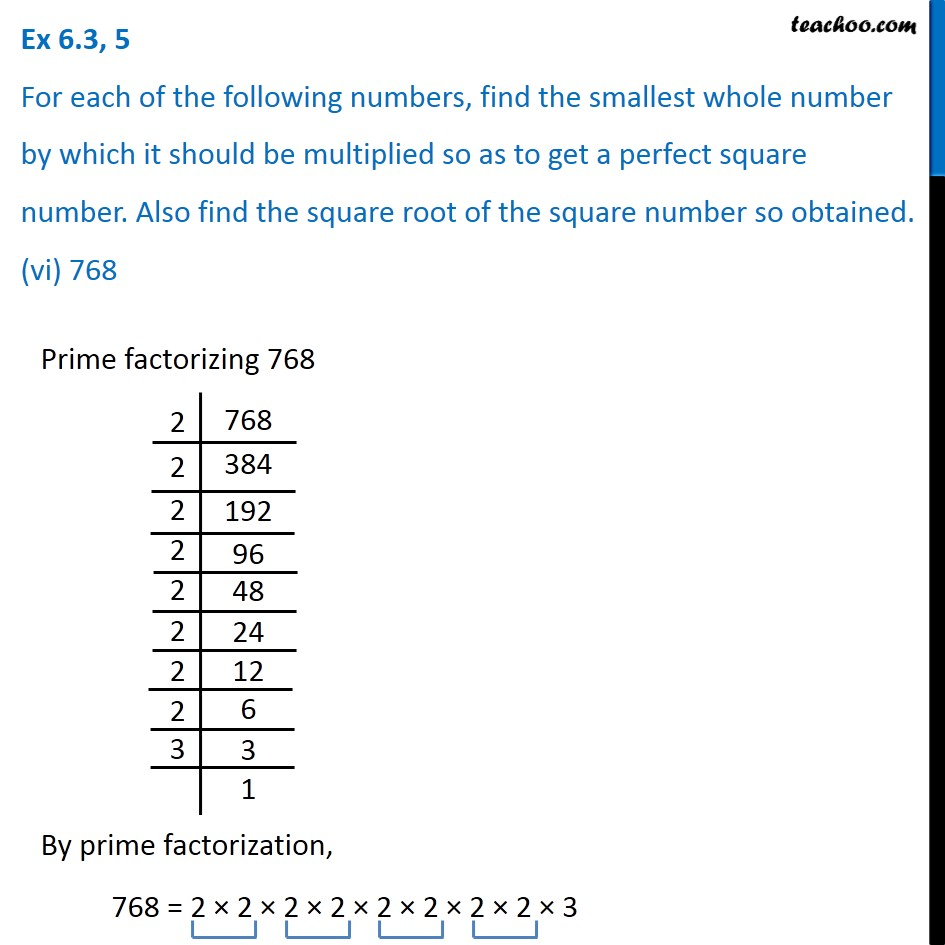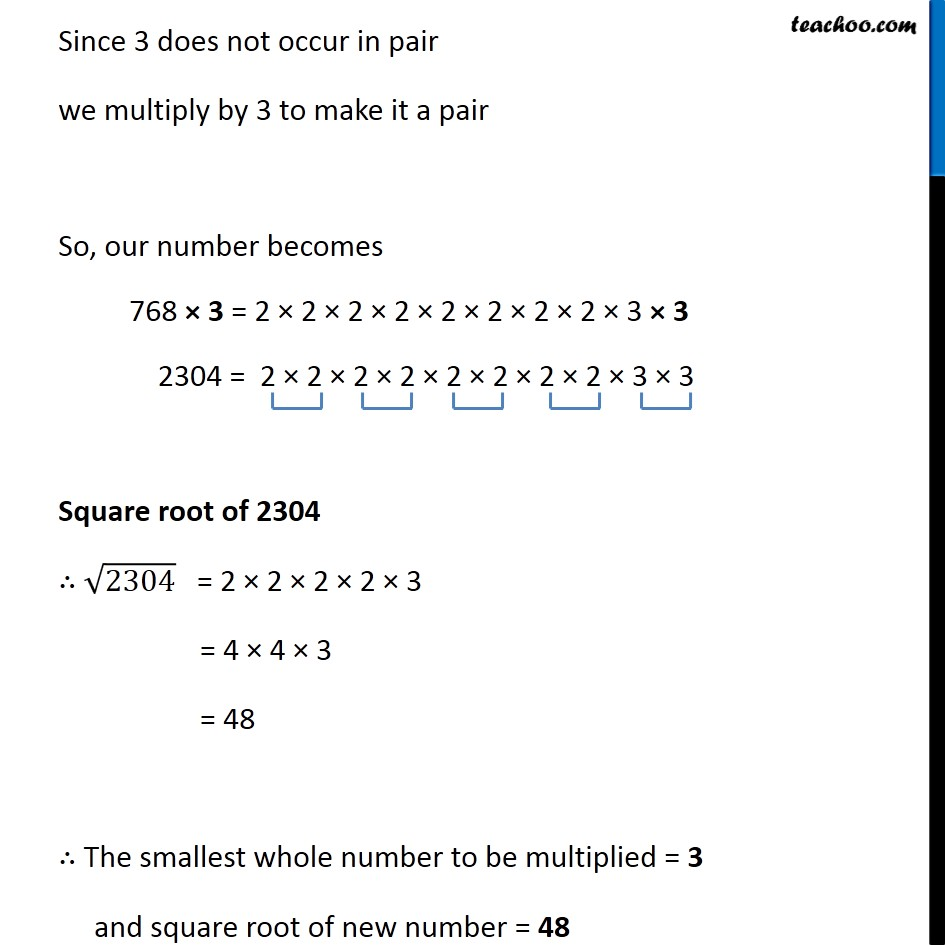Ex 5.3

Chapter 5 Class 8 Squares and Square Roots
Serial order wiseLearn in your speed, with individual attention - Teachoo Maths 1-on-1 Class

### Transcript

Ex 5.3, 5 For each of the following numbers, find the smallest whole number by which it should be multiplied so as to get a perfect square number. Also find the square root of the square number so obtained. (vi) 768 Prime factorizing 768 By prime factorization, 768 = 2 × 2 × 2 × 2 × 2 × 2 × 2 × 2 × 3 Since 3 does not occur in pair we multiply by 3 to make it a pair So, our number becomes 768 × 3 = 2 × 2 × 2 × 2 × 2 × 2 × 2 × 2 × 3 × 3 2304 = 2 × 2 × 2 × 2 × 2 × 2 × 2 × 2 × 3 × 3 Square root of 2304 ∴ √2304 = 2 × 2 × 2 × 2 × 3 = 4 × 4 × 3 = 48 ∴ The smallest whole number to be multiplied = 3 and square root of new number = 48